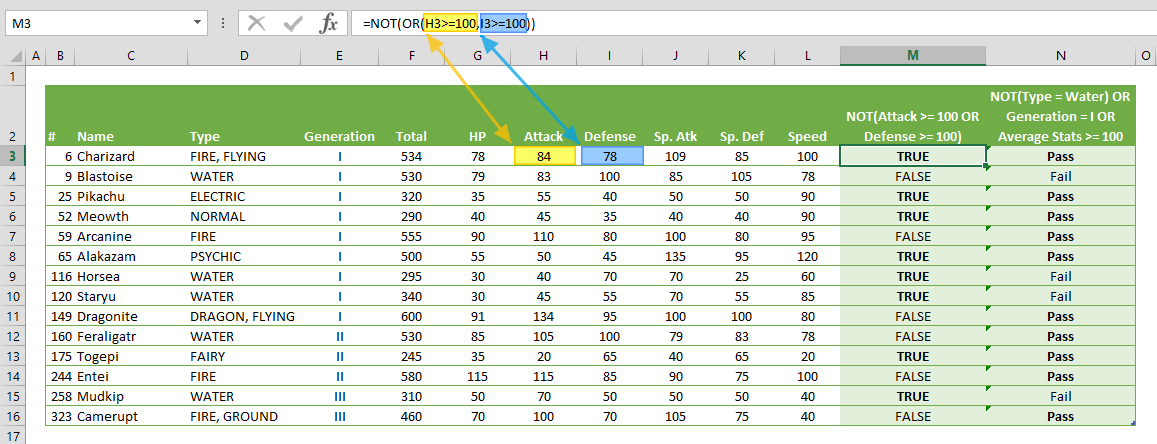The NOT function is a logical function that returns the opposite of its argument. In this guide, we’re going to show you how to use the NOT formula and also go over some tips and error handling methods.

# Supported versions

• All Excel versions

NOT(logical)

# Arguments

 logical A value or expression that can be evaluated as TRUE or FALSE.

Examples

## Example 1

=NOT(OR(84>=100,78>=100))
formula evaluates each test case, and returns TRUE in this example, since the OR function returns TRUE.## Example 2

=IF(OR(NOT("FIRE, FLYING"="Water"),"I"="II",AVERAGE({78,84,78,109,85,100})>=100),"Pass","Fail")
returns Pass because the first test (NOT("FIRE, FLYING"="Water")) returns TRUE. Although the two strings being compared are not the same, the NOT formula reverts the result of the logical statement.# Tips

• Cheat sheet
 Calculation Returns NOT TRUE FALSE NOT FALSE TRUE
• Combine with other logical functions (AND, OR, XOR) to generate more complex scenarios.

# Issues

## #VALUE!

The NOT formula returns #VALUE! if an argument doesn't provide a Boolean value.

## Empty cells

Empty cells are ignored by the formula.